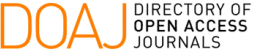### Linear Algebra and Differential Calculus in Pseudo-Intervals Vector Space

Abdelouahab Kenoufi

#### Abstract

In this paper one proposes to use a new approach of interval arithmetic, the so-called pseudo- intervals [1, 5, 13]. It uses a construction which is more canonical and based on the semi-group completion into the group, and it allows to build a Banach vector space. This is achieved by embedding the vector space into free algebra of dimensions higher than 4. It permits to perform linear algebra and differential calculus with pseudo-intervals. Some numerical applications for interval matrix eigenmode calculation, inversion and function minimization are exhibited for simple examples.

PDF

#### References

B. Durand, A. Kenoufi & J.F. Osselin. System adjustments for targeted performances combining symbolic regression and set inversion. Inverse problems for science and engineering, (2013).

A. Trepat, E. Gardenes & H. Mielgo. Modal intervals: Reason and ground semantics. Lecture Notes in Computer Science, Springer-Verlag, Berlin, Heidelberg, 212 (1986), 27–35.

L. Jorba, R. Calm, R. Estela, H. Mielgo, A. Trepat, E. Gardenes & M.A. Sainz. Modal intervals. Reliable Computing, 7 (2001), 77–111.

E. Gardenes. Fundamentals of sigla, an interval computing system over the completed set of intervals. Computing, 24 (1980), 161–179.

N. Goze. PhD thesis, n-ary algebras and interval arithmetics. University of Haute-Alsace, (2011).

A.S. Householder. The theory of matrices in numerical analysis. Dover Publications, (1975).

http://www.ti3.tu-harburg.de/rump/intlab/.

E. Kaucher. Ueber metrische und algebraische Eigenschaften einiger beim numerischen Rechnen auftretender Raume. Dissertation, Universitaet Karlsruhe, (1973).

E. Kaucher. Algebraische erweiterungen der intervallrechnung unter erhaltung der ordnungs und ver- bandstrukturen computing. 1 (1977), 65–79.

E. Kaucher. Ueber eigenschaften und en der anwendungsmoeglichkeiten der erweiterten intervall- rechnung und des hyperbolischen fastkoerpers ueber r computing. 1 (1977), 81–94.

E. Kaucher. Interval analysis in the extended interval space ir computing. 2 (1980), 33–49.

R.B. Kearfott. Rigorous Global Search: Continuous Problems. Academic Publishers, (1996).

A. Kenoufi. Probabilist set inversion using pseudo-intervals arithmetic. Trends in Applied and Com- putational Mathematics, 15(1) (2014), 97–117.

O. Didrit, L. Jaulin, M. Kieffer & E. Walter. Introduction to interval Analysis. SIAM 2009, Applied interval Analysis. Springer-Verlag, London, (2001).

S.M. Markov. Extended interval arithmetic involving infinite intervals. Mathematica Balkanica, New Series, 6(3) (1992), 269–304.

S.M. Markov. On directed interval arithmetic and its applications. J. UCS, 1(7) (1995), 510–521.

S.M. Markov. On the Foundations of Interval Arithmetic. Scientific Computing and Validated Numerics Akademie Verlag, Berlin, (1996).

M. Markov. Isomorphic embeddings of abstract interval systems. Reliable Computing, (3) (1997), 199–207.

http://maxima.sourceforge.net.

R.E. Moore. PhD thesis. Standford university, (1962).

R.E. Moore. Interval analysis. Prentice Hall, Englewood Cliffs, NJ, (1966).

R.E. Moore. A test for existence of solutions to nonlinear systems. SIAM J. Numer. Anal., 14(4) (1977), 611–615.

E. Popova, N. Dimitrova & S.M. Markov. Extended Interval arithmetic: New Results and Applica- tions. Computer Arithmetic and Enclosure Methods. Elsevier Sci. Publishers B.V., (1992).

H.J. Ortolf. Eine verallgemeinerung der intervallarithmetik. geselschaft fuer mathematik und daten- verarbeitung. Bonn, 11 (1969), 1–71.

E.D. Popova. All about generalized interval distributive relations. i. complete proof of the relations. Sofia, (2000).

E.D. Popova. Multiplication distributivity of proper and improper intervals. Reliable Computing, 7(2) (2001), 129–140.

Yves Deville, Pascal Van Hentenryck & Laurent Michel. Numerica: A Modelling Language for Global Optimization. MIT Press, (1997).

http://www.python.org.

C.T. Yang & R.E. Moore. Interval analysis i. LMSD-285875, Lockheed Aircraft Corporation, Missiles and Space Division, Sunnyvale, California, (1959).

Wayman Strother, R.E. Moore & C.T. Yang. Interval integrals. LMSD-703073, Lockheed Aircraft Corporation, Missiles and Space Division, Sunnyvale, California, IX(4) (1960), 241–245.

http://www.sagemath.org.

T. Sunaga. Theory of an interval Algebra and its Application to Numerical Analysis. Number 2. RAAG Memoirs, (1958).

Mieczyslaw Warmus. Calculus of approximations. Bull. Acad. Pol. Sci., C1, III(IV (5)) (1956), 253– 259.

Mieczyslaw Warmus. Approximations and inequalities in the calculus of approximations. classifica- tion of approximate numbers. Bull. Acad. Pol. Sci. math. astr. & phys., IX(4) (1961), 241–245.

W.T. Vetterling, B.P. Flannery, W.H. Press & S.A. Teukolsky. Numerical Recipes in C: The Art of Scientific Computing, 2nd Ed. Cambridge University Press, New York, (1992).

DOI: https://doi.org/10.5540/tema.2016.017.03.0283

### Refbacks

• There are currently no refbacks.

Trends in Computational and Applied Mathematics

A publication of the Brazilian Society of Applied and Computational Mathematics (SBMAC)

Indexed in: